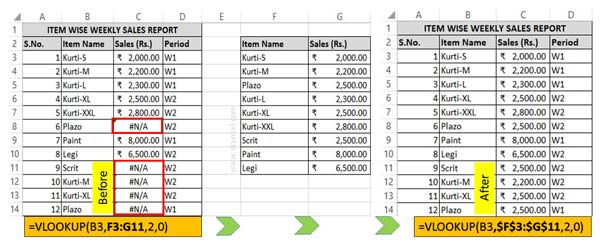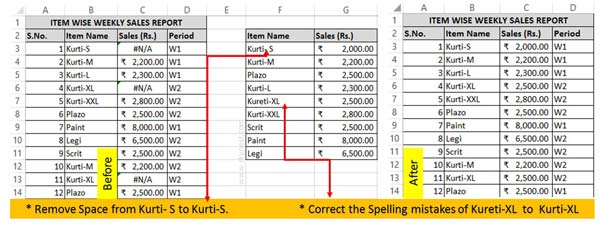# Reason Why VLookup #N/A error shown in Excel

#N/A i.e."not available" when Excel cannot find a lookup value. There are few specific reasons due to which VLookup #N/A error shown.

There are different reasons due to which error values are shown in active worksheet in Excel. The #N/A error values is one of them. There are few important reason due to which VLookup #N/A error shown in Excel sheet.

Excel display different kind of Excel error values like #DIV/0, #N/A, #NAME?, #NULL!, #NUM!, #REF! and #VALUE!. There are different specific reasons due to which these error values in Excel are generated. You can take some precautions or edit the formula so that you can easily resolve the issue of these errors in Excel.

## Reason Why VLookup #N/A error shown in Excel

#N/A error value mans that there is no value available. Normally this is not an error value but it is a special type of value. This kind of error values generated when given formula can’t able to find what it’s been asked to lookup. Normally #N/A error values generated with VLookup, HLookup, Lookup and Match functions when given formula not able to find the given referenced value. There are two common reasons due to which VLookup #N/A error show in Excel sheet, let’s start the discussion.

### First Common reason why #N/A happens with VLookup in Excel

During working in any worksheet, sometime you will get #N/A error value when you are using VLookup function in active worksheet. The reason behind this error values is that after applying the VLookup formula in one cell when you drag the formula down to rest of the cells the table is not correctly referenced. In that situation #N/A error values generated in active worksheet.

If you want to resolve this kind of error value this you must have to check and update the reference type into Absolute reference to solve the issue. All we know there are 3 type reference type which is used in Excel formula- Relative, Absolute & Mixed. Normally Relative reference is used in different formulas but if you try to copy or drag the formula to rest of the cells generate the error values.Step 1: Create the following given table in your active worksheet.

Step 2: Type the following VLookup function in cell C3 =VLOOKUP(B3,F3:G11,2,0) and drag this formula for rest of the cells C3:C14. Now when you drag this formula you can check there are few item names shown #N/A error value in C column.

Step 3: If you want to resolve this type of error then press F2 on cell C3 or you can also double click on Cell C3 to edit the formula.

Step 4: Change the range reference type from relative to absolute like this =VLOOKUP(B3,\$F\$3:\$G\$11,2,0) and drag this formula to rest of the cells. To change the reference type you can use F4 shortcut key or you can also do this job by manually typing the dollar (\$) sign.

### Second Common reason why #N/A happens with VLookup in Excel

If you already change the reference type of given formula but #N/A error values is still show in active worksheet. In that situation you have to check both Lookup and reference value. If both values doesn’t match then you have to check and correct the spelling mistake or remove any extra spaces.Step 1: Create the following given table in your active worksheet.

Step 2: Type the following VLookup function in cell C3 =VLOOKUP(B3,\$F\$3:\$G\$11,2,0) and drag this formula for rest of the cells C3:C14. Now when you drag this formula you can check there are few item names shown #N/A error value in C column.

Step 3: Remove the extra single space from cell F3 and also correct the spelling mistake in Cell F7. Now, you can check #N/A error value has been removed and you will get final resulted value in the respective cells.

I hope after reading this guide you can easily understand what is the exact reasons why VLookup #N/A error shown in Excel sheet. If you have any suggestion regarding this guide then please write us in the comment box. Thanks to all.